# One-Frame Views of Unidirectional Motion

[Background] [Calculator] [ChasePlane] [Example] [1D-Slide] [3D-Slide] [Dynamics]

Caution: This single-frame approach provides students with quantitative tools for dealing with time dilation, relativistic acceleration, and even curved space-time. However, only a multi-frame approach can fully protect students against everyday-language confusion regarding other practical matters manifest at high speeds, like frame-dependent simultaneity and length contraction. For students with only single-frame perspectives on space-time, one bit of advice therefore is to stick to only distances measured using map-frame yardsticks.

Begin with a "map frame", across which is distributed a set of co-moving yardsticks and synchronized clocks. Simultaneity throughout this page will be defined in the context of this single frame of motion. Nonetheless, clocks carried by observers moving with respect to the map frame (i.e. travelers) will keep their own time. Metric equations are taylor-made* for this type of asymmetric situation...

Minkowski's space-time version of Pythagoras' Theorem (the flat-space metric equation) for frame-invariant proper (here "traveler") time is: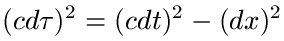or
```(c*Traveler_Time_Elapsed)^2 = (c*Map_Time_Elapsed)^2 - Map_Distance_Traveled^2```

Useful velocity or rate measures that arise because time's passage is frame-variant (i.e. everyone's clocks tell a different story) include...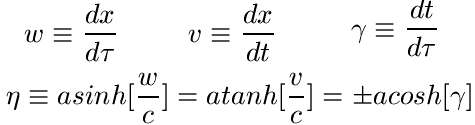or
```Proper_Velocity = Distance_Traveled/Traveler_Time_Elapsed```
```Coordinate_Velocity = Distance_Traveled/Map_Time_Elapsed```
```Speed_of_Map_Time = Map_Time_Elapsed/Traveler_Time_Elapsed```
```Rapidity = ArcSinh[Proper_Velocity/c] = ArcTanh[Coordinate_Velocity/c] = (+/-)ArcCosh[Speed_of_Map_Time]```

From the metric equation, one can obtain the last two equivalences above, as well as the Velocity Conversions below: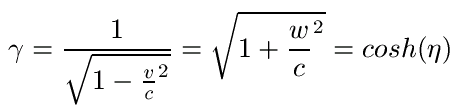or
```Speed_of_Map_Time = 1/Sqrt[1-(Coordinate_Velocity/c)^2] = Sqrt[1+(Proper_Velocity/c)^2] = Cosh[Rapidity]```

Concerning rates of velocity change, the metric equation (along with the metric relation between second proper-time derivatives of map-coordinates) allows us to define frame-invariant proper (felt) acceleration, coordinate (frame-variant) acceleration a, and the relation between them as well: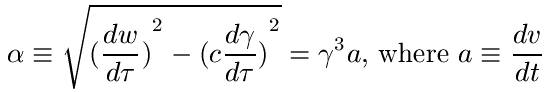or
```Proper_Acceleration = Speed_of_Map_Time^3*Coordinate_Acceleration```

Lastly, the metric equation yields the Motion Integrals of Constant Proper Acceleration: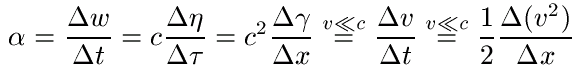or
```Proper_Acceleration = (Final_Proper_Velocity - Initial_Proper_Velocity)/Map_Time_Elapsed```
```Proper_Acceleration = c*(Final_Rapidity - Initial_Rapidity)/Traveler_Time_Elapsed```
```Proper_Acceleration = c^2*(Final_Speed_of_Map_Time - Initial_Speed_of_Map_Time)/Map_Distance_Traveled```

These integrals can be combined to predict changes in any of these variables, in terms of changes in another. For example, a displacement-time equation for constant proper acceleration may be obtained by combining the Rapidity and Speed_of_Map_Time Integrals above: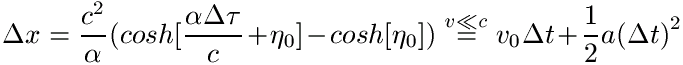On the right of the two equations above, you'll note that these integrals reduce to the simpler Galilean integrals of constant acceleration, when Coordinate_Velocity is much less than Light_Speed c.

Concerning insight into the causes of motion, experiment further provides evidence for the below dynamically conserved quantities which likewise depend on one's frame of reference: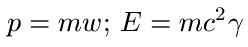or
```Momentum = Mass*Proper_Velocity; Mass_Energy = Mass*c^2*Speed_of_Map_Time```

* no pun intended, even though the metric equation plays a central role in much of Edwin Taylor's recent work.

## JavaScript Constant Proper-Acceleration Calculator

Reconcile yourself to simultaneity as manifest to map-frame observers, specify alternate values for the three green variables below, and then click:

[CALCULATE]

 Differences,Constants\Varying Rates... Initial Value Final Value Map Time Elapsed: [y] Coord. Velocity: [ly/y] Coord. Velocity: [ly/y] Trav. Time Elapsed: [ty] Proper Velocity: [ly/ty] Proper Velocity: [ly/ty] Map Distance Traveled: [ly] Speed of Map Time: [y/ty] Speed of Map Time: [y/ty] Hyperbolic Velocity Angles... Rapidity: [i-radians] Rapidity: [i-radians] Proper Acceleration: [ly/y^2]** Coord. Acceleration: [ly/y^2] Coord. Acceleration: [ly/y^2]

Unit abbreviations on this page: [y]=map_year, [ly]=map_lightyear (a measure of distance), [ty]=traveler_year.
Also: Note that lightspeed c = 1 [ly/y] ~ 3e8 [m/s], and an acceleration of 1[gee] ~ 9.8 [m/s^2] ~ 1.03[ly/y^2].
** The map-frame alone doesn't specify "ownership" of frame-invariant proper acceleration's units (which turn out to be [tly/ty^2]).

## Fashion in Slow-Moving Worlds

A useful curiosity at this point is that it's possible to find a moving observation platform in (3+1)D space-time, which we refer to here as the "Galilean chase plane", from whose vantage point Galileo's classical equations of constant acceleration describe our accelerating traveler's motion at any speed (Way to go, Galileo!). In other words, Proper_Acceleration is the second derivative of Map_Distance_Traveled with respect to local (i.e. Chase_Plane) time. Galilean_Velocity, defined as the first derivative of the Map_Distance_Traveled (by our traveling object) with respect to Chase_Plane_Time, is simply related to the velocities defined above, e.g. via the Energy/mc^2 expression...

`Speed_Of_Map_Time = 1 + (1/2)(Galilean_Velocity/c)^2.`

The chase-plane's state of motion is intermediate to that of traveler and map frames. We don't consider the chase-plane's state of motion further here, even though its simple description of traveler motion is exact at any speed, because the primary utility of chase-plane equations stems from their ease of use (in comparison to above equations involving map and traveler times) when velocities are very small compared to lightspeed c. In this limit, all three times (map, chaseplane, and traveler) are essentially the same, but the Galilean equations are simpler and do not involve small differences between squares. These equations are hence much less sensitive to calculator round-off error, and quite popular in classrooms peopled by sub-relativistic organisms***.

 ChasePlane Time Elapsed: [gy] Galilean V-Initial: [ly/gy] Galilean V-Final: [ly/gy]

*** The rate-focus of sub-relativistic organisms on earth even at high speeds has for historical reasons been primarily on Coordinate_Velocity and Gamma (Speed_of_Map_Time). Some of the other rates discussed here might warrant attention as well. For example, Coordinate_Velocity compresses the entire range of relativistic energies into a tiny span of velocities just below 1 [ly/y] (e.g. making it more difficult to impress voters with particle accelerators that attain new "land speed records" with their projectiles). On the other hand Proper_Velocity is directly proportional to momentum, is part of an easily transformed 4-vector, and obeys a simpler velocity-addition rule (since one combines two values of Proper_Velocity = Speed_Of_Map_Time * Coordinate_Velocity by adding Coordinate_Velocities then multiplying by the Speeds_Of_Map_Time). Proper_Velocity also highlights a natural symmetry in log space between sub-relativistic and relativistic regimes, about the value of 1 [ly/ty] (which for culture-specific mnemonic purposes, and perhaps ironically because the StarTrek series did such a poor job of recognizing the lightspeed limit, some here glibly refer to as "a roddenberry"), and lessens temptation to introduce the anachronistic concept of relativistic mass (assuming that few relish the idea of a mass which varies with direction, when motion ceases to be unidirectional).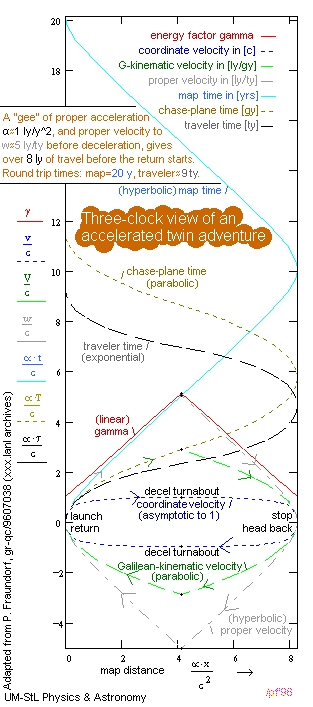## A Worked Example...

This seven versus one variable plot, predicated on simultaneity defined by the perspective of a starting-point reference "map-frame" of coordinate axes and synchronized clocks, illustrates the above relationships for a 16.5 lightyear round-trip "accelerated twin" adventure involving four constant Proper_Acceleration segments (accelerate away, decelerate to a stop, accelerate to return, decelerate to stop) each lasting about 5 map-years or about 2.27 traveler-years. Plotted as a function of Map_Distance x, note the hyperbolic nature of Map_Time, the much slower progress of Traveler_Time in terms of which Map_Distance is a sum of exponentials, and the intermediate parabolic nature of elapsed-time as measured by the Galilean chaseplane. As Map_Distance increases, also note the linear behavior of gamma (the Speed_of_Map_Time as far as traveler clocks are concerned), the hyperbolic nature of Traveler_Velocity, the asymptotic nature of Coordinate_Velocity, and the intermediate parabolic nature of chaseplane-mediated Galilean_Velocity.

Equations for each of these quantities, in a given segment of the trip, are quite easy to obtain from the integrals of constant Proper_Acceleration given above. Thus with the right travel arrangements, you can find out how your younger sibling behaves when they find themselves senior to you by ten years! There is risk, of course, because if you don't like the way they behave, it may not be possible to go back to being the older of the two, unless you can persuade them to take a similar trip.

This page is http://www.umsl.edu/~fraundor/a1djs.html. Although there are many contributors, the person responsible for errors is P. Fraundorf. This site is hosted by the Department of Physics and Astronomy (and Center for Molecular Electronics) at UM-StL. For more on one-frame views of space-time, check out our table of contents. Older proper-acceleration calculators can be found here, here, and here. A nano-microscopy field calculator may be found here. Whole-site page requests est. around 2000/day hence more than 500,000/year. Requests for a "stat-counter linked subset of pages" since 4/7/2005: .